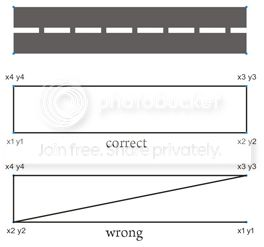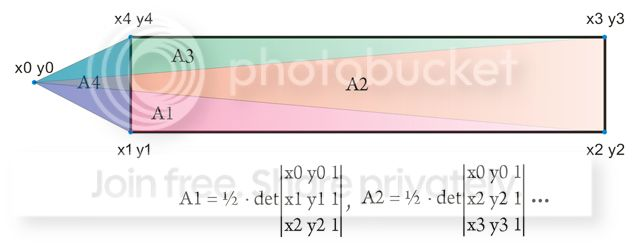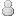Register Blogs Orbinauts List Social Groups FAQ Projects Mark Forums Read

Rate this Entry

# A fun ride in math-land

Posted 12-23-2012 at 03:43 PM by dgatsoulis
Updated 12-24-2012 at 11:17 PM by dgatsoulis

Ok, this was a fun ride and I wanted to share it with you guys.

It started with a "simple" question in the OrbiterSDK forum, where I asked how to determine if a vessel is on a runway or outside of it.
The solution is trivial for a runway aligned with x and y (East-West or North-South) but what happens in other cases?

Quote:
What about defining the runway with barycentric coordinates?
A few minutes later RisingFury jumped in. He had solved exactly that problem for OBSP so he handed me the C++ code and the explanation.

I understood RisingFury's solution for the problem and I was able to apply it. Basically it's using trigonometry to get the define a triangle whose sides are the distances of the vessel from endpoint1 & endpoint2 of the runway and the 3rd side is the length of the runway. Then you find the angles of the triangle and combined with the runway width, one is able to determine whether a vessel is inside it or not.
My "Trig-Fu" was never very strong, but the equations and the explanation were handed to me, so it was relatively easy to apply it in Lua script.

But I was intrigued by Urwumpe's suggestion. All I knew was what a barycenter is and that's where my knowledge ended. So, I looked up "barycentric coordinates" and the wiki page looked very interesting to me. Perhaps because I always prefered algebra to trigonometry.

If I could do this, I would be able to define a rectangular area with two triangles and then determine if a point with coordinates x and y is inside those triangles or not.
In fact, one can choose any 2D area, find it's barycenter, break it down to triangles and apply the same method.
I had no idea how I would be able to do it; plus my math skills are at highschool level - which I graduated from almost 20 years ago.

But that's no reason to quit. I have a good understanding of basic algebra and as long as things don't get too complicated too fast, I am usually able to follow what I'm reading.

I searched for "point inside a rectangle" and I found a few very interesting methods. The one that looked the "simplest" and more elegant to me was this one from the math forums:

Quote:
Given any three points on the plane (x0,y0), (x1,y1), and
(x2,y2), the area of the triangle determined by them is
given by the following formula:To be inside a rectangle (or any convex body), as you trace
around in a counter-clockwise direction from p1 to p2 to p3 to p4 and
back to p1, the "areas" of triangles p1 p2 p, p2 p3 p, p3 p4 p,
and p4 p1 p must all be positive. If you don't know the
orientation of your rectangle, then they must either all be
positive or all be negative."
This is exactly what I thought:Joking aside, I could almost understand this. I had to define the "points" that outline a runway, calculate the areas of the triangles that are defined by my vessel and two of those "points" and then if all the areas are positive (or all negative), the vessel is inside. Otherwise, it's outside.

But I didn't know what a determinant was, so I looked it up.
Basically, for a 3x3 table, it's like trying to figure out how to write "123" in all possible combinations: 123 132 213 231 321 312. Then you start with + - and you alternate them:
+123-132-213+231+321-312.
Each number "place" represents the "row" in the table and the number itself represents the "column". (Don't ask me about the signs, I don't know why you place them in that order -yet).

So now, it was easy to convert the formula for the area of a triangle into something I could understand more easily:At this point I thought: "Oh crap, now I'll get lost with all those "x1"s and "y2"s and it will get very complicated as I add more points to define the area that I want."
Still, I wanted to follow this through and see were it would take me, (or at which point I would give up).

So I got my self some (electronic) pen and paper and traced a rectangle along "counter-clockwise-ly". I made sure that I wouldn't mix the order of the points.Then moved the mouse to a random point on the screen. That would be my vessel with x0y0 coordinates. I also colored the areas of the triangles to see it better.Then I re-wrote the equation for A1 and started to write the equation for A2 below it.
As I looked at it I realised that it wasn't complicated after all. To get the equation of the next area, all I had to do was to copy/paste, leave x0 and y0 the same and "go up one" in the other "x"s and "y"s. (This will make more sense when you see all 4 equations and follow the "x"s and "y"s vertically).That's all I needed. Now all I had to do was to check if the values of all the areas where positive, or if they were all negative. That would mean that the vessel is inside. Ortherwise, it would be outside.

Coding it in Lua was a breeze:

Code:
```
x1 = -135.473650
x2 = -135.38672
x3 = x2
x4 = x1
y1 = 12.744590
y2 = y1
y3 = 12.746220
y4 = y3 -- finished outlining the area. This one is aligned with x and y but it could be any shape really
hobj = v:get_surfaceref()
equ = oapi.global_to_equ (hobj, v:get_globalpos())
x0 = equ.lng*180/PI
y0 = equ.lat*180/PI -- got x and y of my vessel
a_1 = 0.5*((x1*y2)-(y1*x2)-(x0*y2)+(y0*x2)+(x0*y1)-(y0*x1))
a_2 = 0.5*((x2*y3)-(y2*x3)-(x0*y3)+(y0*x3)+(x0*y2)-(y0*x2))
a_3 = 0.5*((x3*y4)-(y3*x4)-(x0*y4)+(y0*x4)+(x0*y3)-(y0*x3))
a_4 = 0.5*((x4*y1)-(y4*x1)-(x0*y1)+(y0*x1)+(x0*y4)-(y0*x4)) --got the areas, now all I need is to check if all are positive
vessel_inside=0
if a_1 > 0 and a_2 > 0 and a_3 > 0 and a_4 > 0 then
vessel_inside=1
end
if a_1 < 0 and a_2 < 0 and a_3 < 0 and a_4 < 0 then
vessel_inside=1 -- Just in case I moved clockwise while going through the points that outline the area
end```
I tested it and it worked beautifully.
The great thing is that you can add as many points XnYn as you want. You simply copy paste from the previous calculation for the area, leave x0 and y0 as they are and "go up" a value in the rest "x"s and "y"s. You just have to make sure that to get to the next point, you trace the perimeter of the area you want in a counter-clockwise manner.

Now I'm pretty sure that there must be an even easier solution for this problem, but for me it was a quite a fun journey of exploration in "unknown" territory. All it took was some paper, a pen and a couple of hours of my time.

I guess the moral of the story is: simply because something is "unknown", doesn't mean it's "unknowable" and things that might seem complicated at first glance, may turn out to be simple if you take some time to investigate them.

Many thanks to Urwumpe and RisingFury for helping me with my question.
Posted in Uncategorized

1.Well doneYou've certainly learned some math, but the more important bit you've learned here is to find the solution to your problem through to the end. Most people are so afraid of math that they'll usually do anything to avoid it. I do have a question, though. Run a test with a runway that has a heading of 45°, so not exactly north-south or east-west. Check what happens at all of the edges of the runway. Is the algorithm still accurate there? I'm guessing there should be some inaccuracy due to the fact you're using 2D math on a 3D body. I remember I run into that error when making the bomb landing prediction function for OBSP. It predicted that the bomb would land left or right of the aircraft, which was complete nonsense. I never measured the error, but it was enough to see it with the naked eye, so I'm guessing it was within the few 10 meter range. Do your test with a 3 to 5 km long runway and let me know what happens, pleaseKeep up the good workP.S.: I hate trig too, but it sure is useful when navigating on a sphere. Posted 12-23-2012 at 06:47 PM by RisingFury2.The math should work out also for strange angles or even shapes that are not rectangular. The test that all areas have the same sign could be simplified if you use an order of the vertexes that can only be negative if the fourth point is outside the triangle. You should get problems at the poles, where you could get errors in the projection. But you could use a 90° rotated projection from spherical to cartesian coordinates if the runway is at a latitude beyond 80° N/S. Posted 12-23-2012 at 06:56 PM by Urwumpe3.Quote: Originally Posted by RisingFury Well done ...{snip}... I do have a question, though. Run a test with a runway that has a heading of 45°, so not exactly north-south or east-west. Check what happens at all of the edges of the runway. Is the algorithm still accurate there? Thanks The coordinates in the example were for a 5km runway on Mars with a 90/270 direction. In my test, there was no noticable/visible error in defining if the vessel was inside or outside. But as you can see it was at Olympus Base, which is pretty close to the equator (12.74°N) and I used 5 decimals in each of the corner's coordinates. I'll do some testing on Earth and also try it out at the meridian and the equator with 45° angle and try some random odd-shaped 2D areas as well. I'll get back to you with the results. Quote: Originally Posted by Urwumpe The test that all areas have the same sign could be simplified if you use an order of the vertexes that can only be negative if the fourth point is outside the triangle. Yeah, it's really simple. You can pic any point and call that x1y1. Then if you trace the 2D area by moving to the next point in a counterclockwise direction, the areas will always be positive if you are inside. Quote: Originally Posted by Urwumpe You should get problems at the poles, where you could get errors in the projection. But you could use a 90° rotated projection from spherical to cartesian coordinates if the runway is at a latitude beyond 80° N/S. Good call. I don't think it should matter, because I'm using the same coordinates for the vessel and the 4 points but I'll setup a couple of runways to see what happens at high lattitude. Posted 12-23-2012 at 07:39 PM by dgatsoulisUpdated 12-23-2012 at 07:46 PM by dgatsoulis
4.try it with a runway directly at the south pole. it could get funny. Posted 12-23-2012 at 07:44 PM by Urwumpe5.Quote: Originally Posted by RisingFury ]Do your test with a 3 to 5 km long runway and let me know what happens, please I tested it and did not find any noticable errors for lattitudes up to 85. At around 87, it started to get visible. I posted an attachment in the OrbiterSDK thread. Next I'll check irregular shapes. Quote: Originally Posted by Urwumpe try it with a runway directly at the south pole. it could get funny. Yes it got very funny as I approached the pole. At exactly -90 the DG was returning me Y values all over the place and it got into NaN space as soon as I fired the engines. Posted 12-24-2012 at 03:18 AM by dgatsoulis6.Finished checking irregular 2D shapes. It works great. Posted the video and the code on the SDK forum, I might as well place it here too. Here is the code: Code: ```-- sanity checks v = vessel.get_interface('GL1') if v == nil then term.out ('Could not find 1. Aborting') return elseif v:get_classname() ~= 'DeltaGlider' then term.out ('Wrong vessel class. Aborting') return end -- set the types of notes position size and color on screen note = oapi.create_annotation() note:set_pos (0.002,0.23,0.5,0.5) note:set_colour ({r=1,g=1,b=0}) note:set_size (0.8) gnote = oapi.create_annotation() gnote:set_pos (0.815,0.23,0.998,0.5) gnote:set_colour ({r=1,g=1,b=0}) gnote:set_size (0.8) -- wait for goals to be completed (I'm using this code for challenges, so I'm keeping this format) contact = false vessel_inside=0 goal2=0 goals=vessel_inside+goal2 while goals < 5 do --arbitrary number larger than the number of goals so the script doesn't close. In my challenge I set this to the number of goals gnote:set_text (string.format (" GOALS\n---------------------------------\n Check If the DeltaGlider is\n on the ground or in the air\n Return Ground or Airborn\n If Ground Contact is true \n check if inside cyan area\n Return Inside or Outside")); vessel_inside=0 --my vessel's coordinates. I need to have them in the same units as the coordinates of the shapes hobj = v:get_surfaceref() equ = oapi.global_to_equ (hobj, v:get_globalpos()) x0 = equ.lng*180/PI y0 = equ.lat*180/PI -- coordinates of the triangle points. important to move counter-clockwise to get to the next point xa1 = 27.997948 xa2 = 28.003588 xa3 = 27.996508 ya1 = 35.998773 ya2 = 35.997393 ya3 = 36.002883 -- areas of the triangles defined by each side of the shape above and my coordinates a_1 = 0.5*((xa1*ya2)-(ya1*xa2)-(x0*ya2)+(y0*xa2)+(x0*ya1)-(y0*xa1)) a_2 = 0.5*((xa2*ya3)-(ya2*xa3)-(x0*ya3)+(y0*xa3)+(x0*ya2)-(y0*xa2)) a_3 = 0.5*((xa3*ya1)-(ya3*xa1)-(x0*ya1)+(y0*xa1)+(x0*ya3)-(y0*xa3)) -- check if all areas are positive if a_1>0 and a_2>0 and a_3>0 then vessel_inside=1 end -- coordinates of the irregular rectangle points. Important to move counter-clockwise to get to the next point xb1 = 28.010138 xb2 = 28.012718 xb3 = 28.010758 xb4 = 28.005328 yb1 = 35.997543 yb2 = 35.997983 yb3 = 36.000623 yb4 = 36.000973 -- areas of the triangles defined by each side of the shape above and my coordinates b_1 = 0.5*((xb1*yb2)-(yb1*xb2)-(x0*yb2)+(y0*xb2)+(x0*yb1)-(y0*xb1)) b_2 = 0.5*((xb2*yb3)-(yb2*xb3)-(x0*yb3)+(y0*xb3)+(x0*yb2)-(y0*xb2)) b_3 = 0.5*((xb3*yb4)-(yb3*xb4)-(x0*yb4)+(y0*xb4)+(x0*yb3)-(y0*xb3)) b_4 = 0.5*((xb4*yb1)-(yb4*xb1)-(x0*yb1)+(y0*xb1)+(x0*yb4)-(y0*xb4)) -- check if all areas are positive if b_1>0 and b_2>0 and b_3>0 and b_4>0 then vessel_inside=1 end -- coordinates of the irregular pentagon points. important to move counter-clockwise to get to the next point xc1 = 28.016268 xc2 = 28.020388 xc3 = 28.021108 xc4 = 28.018698 xc5 = 28.014218 yc1 = 35.997183 yc2 = 35.998113 yc3 = 36.000933 yc4 = 36.002633 yc5 = 36.001333 -- areas of the triangles defined by each side of the shape above and my coordinates c_1 = 0.5*((xc1*yc2)-(yc1*xc2)-(x0*yc2)+(y0*xc2)+(x0*yc1)-(y0*xc1)) c_2 = 0.5*((xc2*yc3)-(yc2*xc3)-(x0*yc3)+(y0*xc3)+(x0*yc2)-(y0*xc2)) c_3 = 0.5*((xc3*yc4)-(yc3*xc4)-(x0*yc4)+(y0*xc4)+(x0*yc3)-(y0*xc3)) c_4 = 0.5*((xc4*yc5)-(yc4*xc5)-(x0*yc5)+(y0*xc5)+(x0*yc4)-(y0*xc4)) c_5 = 0.5*((xc5*yc1)-(yc5*xc1)-(x0*yc1)+(y0*xc1)+(x0*yc5)-(y0*xc5)) -- check if all areas are positive if c_1>0 and c_2>0 and c_3>0 and c_4>0 and c_5>0 then vessel_inside=1 end --msgs and info on screen msg = string.format("-------------------\n Info\n-------------------\n X: %.6f \n Y: %.6f\n-------------------\n Messages\n------------------- ",x0, y0) note:set_text (msg) alt = v:get_altitude() contact = false if contact == false then contact = v:get_groundcontact() if contact == true then msg = msg.."\n Ground" end note:set_text (msg) if alt >= 3 then contact = false msg = msg.."\n Airborn" end end if vessel_inside<1 and contact == true then msg = msg.."\n Outside" end if vessel_inside>0 and contact == true then msg = msg.."\n Inside" end note:set_text (msg) proc.skip() -- for challenges. check if goals have been met and close the script hobj = v:get_surfaceref() planet = v:is_landed() if oapi.get_objname(planet) ~= 'Earth' then planet = nil end goal2=0 if planet ~= nil then goal2=1 end goals=vessel_inside+goal2 if goals > 4 then -- same as at the beginning minus one. I use it for challenges, but here I don't want to close the script end end oapi.del_annotation (gnote)``` Posted 12-26-2012 at 08:54 PM by dgatsoulisAll times are GMT. The time now is 09:46 PM.